# Custom Table Calculations: Creating Expressions

Follow

Custom calculations are built in the expression editor. As you type your expression, the system prompts you with functions, operators, and field names that you might want to use. It works as described below.

### Seeing All Suggestions

Type a space to see a list of all the fields, functions, and operators that you can choose from. It’s quite a long list, so you will typically want to start typing to shorten the list to the items you’re interested in.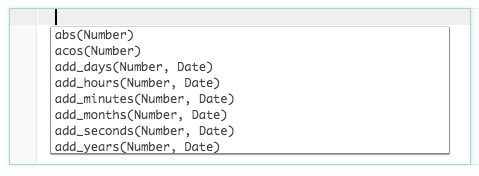To include a field in your expression, start typing the field’s name. As you type, the editor will narrow your search to a list of fields and functions that contain what you’ve typed. When you select a field from the list, system adds it to your expression in the form of `\${view_name.field_name}`. This ensures that all of your fields have unique names in your expression.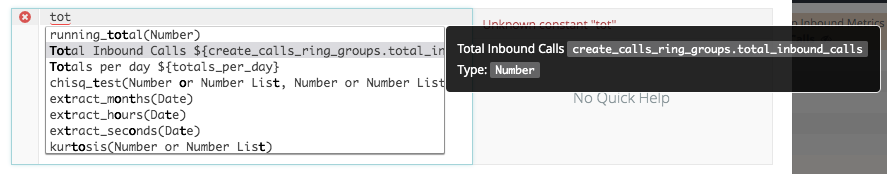You can add logical operators like AND, OR, and NOT to your expression if needed. Ordinarily AND operators are evaluated before OR operators, but you can override this behavior by using parentheses. You also can use comparison operators (such as >, =, and <=) and mathematical operators (such as + and *).

When your cursor is on an operator, you can check the notes that are displayed to the right of your expression in the information pane for proper use.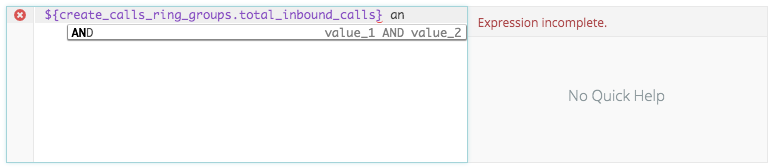To include a function in your expression, start typing the function’s name. As you type the editor will narrow down your search to a list of fields and functions that contain what you’ve typed.

Functions may be constructed of arguments (or variables) that require a certain type, such as a field, a number, or yes/no. When your cursor is on a function, you can check the notes that are displayed to the right of your expression in the information pane to understand which arguments you need to provide, and what type they need to be.

To see the full list of functions check out our Data Dictionary page dedicated to Custom Calculations.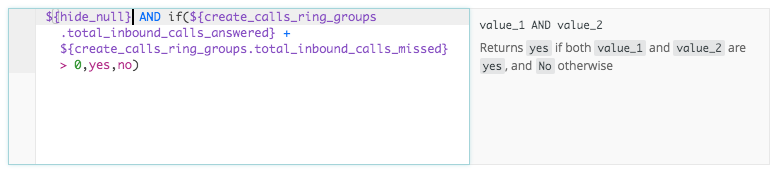### Using Error Hints and the Information Pane

Note that as you type an expression, information is displayed in pane to the right. This pane provides suggestions, especially if you have an error in your expression.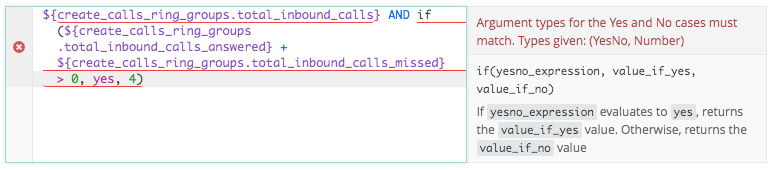As shown above, the editor provides:

• Error Highlighting. In the editor itself, any parts of the expression that are not yet correct will be underlined in red.
• Suggestions and Error Details. The top part of the information pane gives suggestions about what to add next in your expression. If there’s an error, it explains why the error is occurring. If there are multiple errors, the error that it shows to you is based on the location of your cursor.
• Argument AutofillWhile you type the first argument of an `if ()` function, the system provides the information that the first argument should evaluate as true or false.

### Using Fields

Sometimes you’ll want to use the value of a field (a dimension, measure, or custom calculation) in an expression. You might want to add the value of the field to something else, check that it has a certain value, include it in a function, or many other possibilities.

As described above, just type the name of the field into the expression editor, and the system will help you find the correct way to reference it. When you add a field to an expression, the system uses the field’s identifier, which looks like `\${view_name.field_name}`. This identifier does not always match the name of the field in the Field Picker, but it’s okay if you don’t know what it is. Just type the field name as it appears in the Field Picker and the expression editor will show you the Field Picker name and the identifier together.

There are several ways you might want to retrieve a value.

Get a Value from the Same Row

The most basic way to use a field is to reference it directly. For example, your expression might use

`\${product.category}`

When you do this, you’re saying “for any given row, grab the Call Type from that row.”

Get a Value from a Different Row

You can also get a field’s value from a different row. For example, you might want the logic “for any given row, grab the Call Type from the previous row.” To do that, you can use an offset function. It might look like this.

`offset(\${call_type}, -1)`

Get a Value from a Pivoted Column

You can also get values from pivoted columns. For example, you might want the logic “for any given row, grab the Total Calls from the first pivoted column.” To work with pivoted columns, you’ll need to use pivot functions. It might look like this.

`pivot_index(\${total_inbound_calls_answered}, 1)`

Get a Total from a Row or Column

If you added totals to your Explore, you can get total values from the column or row by adding `:total` (for column totals) or `:row_total` (for row totals) to the field name, using the format `\${field_name:total}`. For example, if you want a percentage of the total of a Calls count, you could create a custom calculation like this:

`\${total_inbound_calls_answered} / \${total_inbound_calls_answered:total}`

### Using Operators

Expressions sometimes include logical, comparison, and mathematical operators to help you create different conditions:

• Logical operators are things like AND, OR, and NOT
• Comparison operators are things like > and <
• Mathematical operators are things like + and -

These are usually straightforward to use. Just keep in mind that AND logic is considered before OR logic, unless you specify otherwise with parentheses. Thus the following expression without additional parentheses:

`if (  \${argument.1}>=4 OR  \${argument.2}>5 AND  \${argument.3},"volume up", "volume down")`

would be evaluated as:

`if (  \${argument.1}>=4 OR  (\${argument.2}>5 AND \${argument.3}),"volume up", "volume down")`

The other thing to keep in mind is how true and false is handled. You should use yes and no instead of true and false. These logical constants are not the same thing as the words "yes" and "no", which are enclosed in quotes.

### Using Functions

Expressions often include one or more functions, which help you to retrieve certain data or calculate certain things. They are similar in nature to Excel functions, so if you’ve used Excel functions, you’ll be comfortable with using functions in Explore.

If you are not familiar with functions, they take the form of a name followed by two parentheses, like this:

`my_function()`

Sometimes you’ll need to provide information within those parentheses, separated by commas. These bits of information are called “arguments” and look like this:

`my_function(argument_1, argument_2)`

For example, the now function does not take any arguments, and gives you the current date and time. You’d use it like this:

`now()`

In contrast, the round function does take one argument, which is a number. You’d use it like this.

`round(3.2)`

The result you would get is 3.

There are two ways to know which arguments you’ll need to provide, if any:

1. The information pane that appears to the right of the expression editor will provide some detail about the function you are writing.
2. You can also navigate directly to Functions and Operators page and look up the function you want to use.

To solidify this concept, consider the `contains` function:

 Function Syntax Purpose `contains` `contains(string, search_string)` Returns Yes if string contains search_string, and No otherwise

You can see that two arguments are required. They have the names `string` and `search_string`, but that doesn’t mean you need to type the exact word “string” and “search_string” into the function. These are just names for the arguments that you’ll replace with something. Reading the purpose, we see that string should be a field or other value we want to search in, while the `search_string` is the thing we want to search for. An example might be:

`contains(\${call_direction}, "Inbound") `

If the word “Inbound” appears in the distribution this function will give us a result of Yes, otherwise it will give a result of No. The word that is to be searched and it’s placed within commas is case sensitive, so in this specific case you need to capitalize the initial “I” so that the function recognizes the word and returns the proper results, otherwise all the results would return “No”.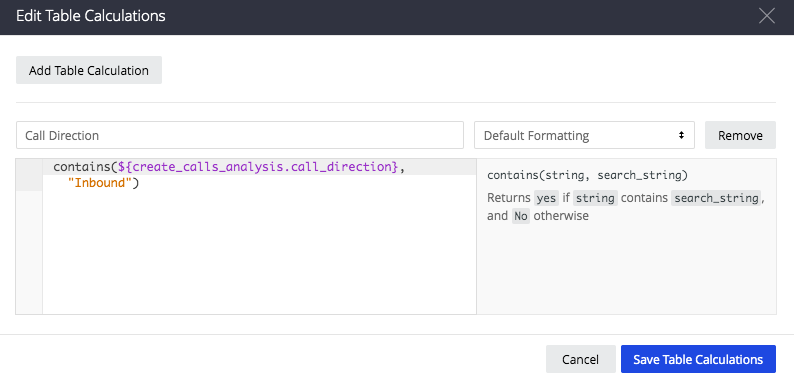The table with results from the Custom Table Calculation would be: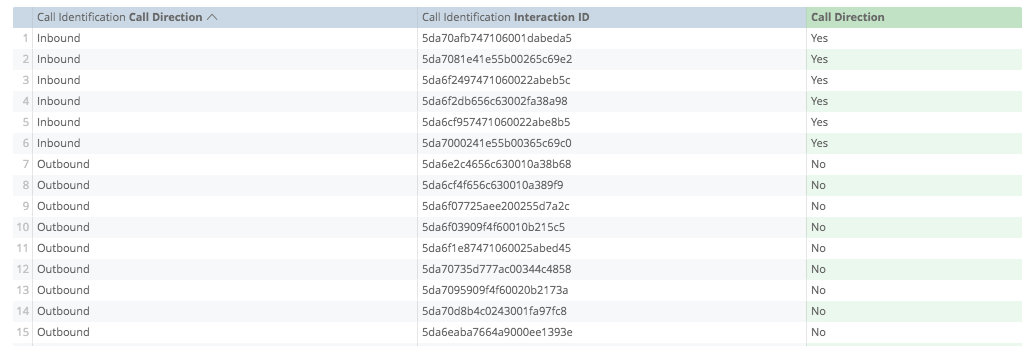The final thing to know is that you can put functions inside other functions to handle whatever complex logic you want. As long as the result of the inner function is appropriate for the arguments of the outer function, it will work. For example:

`mod(diff_days(date(2019,01,01), \${create_calls_ring_groups.datetime}) + 2, 7) `

Here we’ve nested the `date` function inside of `diff_days` function, which is itself inside a mod function. It works like this:

1. The `date()` function checks which date we are considering in our distribution, in this case, `\${create_calls_ring_groups.datetime}`.
2. Next, the `diff_days()` function looks at `start_date` (2019,01,01) and `end_date` (`\${create_calls_ring_groups.datetime}`); and returns the number of days between the 2 (let’s say our end date would be 2019.09.30; the number of days between the 2 dates is 272 days).
3. Finally, the `mod()` function returns the remainder (to the 272 days we’ve added 2, so it considers Monday as day 1 of the week).

The complete calculation would be:

• 274 (the difference of days between start and end date plus 2 days to adjust the first day of the week) by 7 (number of days in a week) to arrive at 39.1428571429 (274/7=39.1428571429).
• Round this to a whole number of 39. Multiply 39 by 7 to achieve 273 (39*7=273).
• Subtract this from the original number of 274 to arrive at a remainder of 1 (274-273=1), which is the ordinal number of Monday on a week for our specific analysis

The Functions and Operators documentation lets you know which functions are the most appropriate to your needs. When using functions in a table calculation you may use any function.